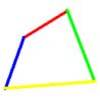#### You may also like### Real(ly) Numbers

If x, y and z are real numbers such that: x + y + z = 5 and xy + yz + zx = 3. What is the largest value that any of the numbers can have?### Biggest Bendy

Four rods are hinged at their ends to form a quadrilateral. How can you maximise its area?### Quartics

Investigate the graphs of y = [1 + (x - t)^2][1 + (x + t^)2] as the parameter t varies.

# Little and Large

##### Age 16 to 18 Challenge Level:
Congratulations to Soh Yong Sheng from Raffles Institution, Singapore for this excellent solution.

We have $0 < a < b$ which means $1/a > 1/b$ and so $3 + 1/a > 3 + 1/b$. Flipping over the fraction, we will get 1/(3 + 1/a) < 1/(3 + 1/b) and the inequality remains the same way round when 2 is added. Flipping over again for the last time we get $\frac{1}{2+\frac{1}{3+\frac{1}{a}}}$ is greater than $\frac{1}{2+\frac{1}{3+\frac{1}{b}}}$

The second part is a further expansion of the first, and in the process of repeating the above we know that it involves just one more flipping over of the fraction, thus $\frac{1}{2+\frac{1}{3+\frac{1}{4 + \frac{1}{a}}}}$ is less than the same thing with $b$ in place of a as the inequality would be reversed again.

Lastly the continued fractions are expanded all the way down to $100 + 1/a$ and $100 + 1/b$. Observe the above process, we can tell that if the last or biggest number is odd then the continued fraction with a in it is bigger. If the last or biggest number is even then the continued fraction with $b$ in it is bigger. Each successive continued fraction involves one more 'flipping over' and reverses the inequality one more time. The following continued fraction is smaller than the same thing with $b$ in place of $a$: $${1\over\displaystyle 2 + { 1 \over \displaystyle 3+ { 1\over \displaystyle 4 + \dots + {1\over\displaystyle 99+ {1\over \displaystyle {100 + {1 \over \displaystyle a}} }}}}}$$